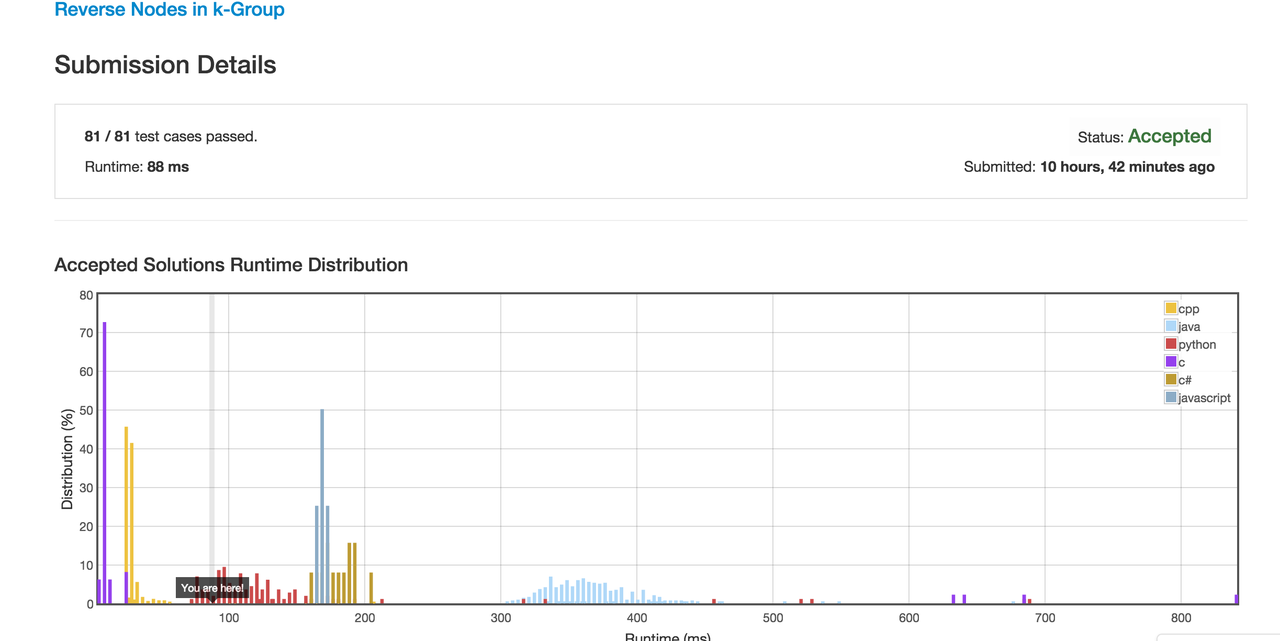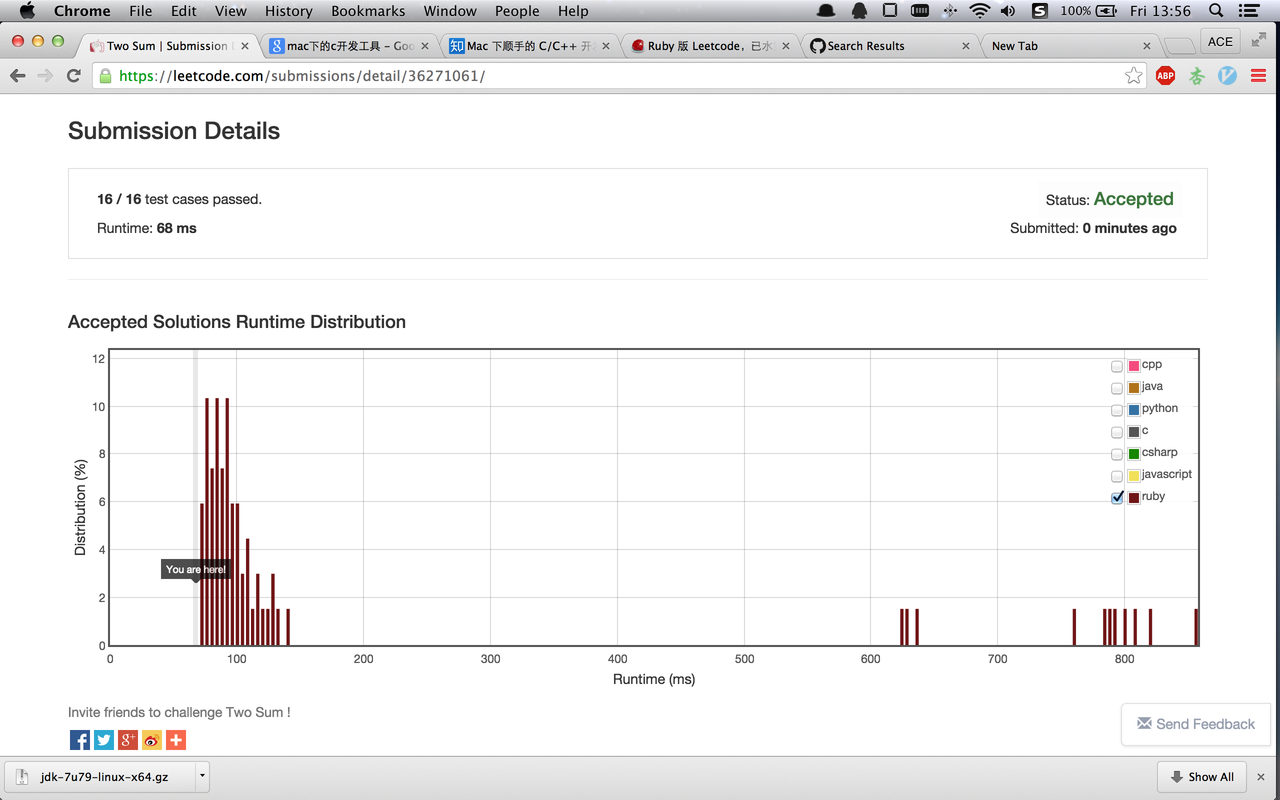# Ruby Ruby 版 Leetcode，已水完 100 题，求同好 Review

arth · 2015年07月17日 · 最后由 steve 回复于 2023年02月03日 · 9200 次阅读

1.确实有开挂的感觉，使用 ruby 的话可以不用关心溢出、字符串数字互转、二进制十进制互转。对于专门要考察这些操作的题不应当使用 ruby 特性解决外，其他问题完全可以利用这种特性；

1. ruby 的负数除法是向负无穷取整的。10/(-3) 得到的是 -4 而不是 -3。这和 c 系语言向零取整恰好相反。这样，c 系语言能过的用例，只要有负数除法，ruby 必然过不了，需要处理一下才能 AC。记得这个问题是在求解逆波兰式时遇到的。
2. 如果题目要求不要返回任何对象，那你需要在方法的最后一行填上 return 空语句。否则 ruby 会把之前循环体或者其他最后的对象返回。
3. 如果需要返回空串，就 return "", return String.new 产生的是个空数组 [ ] 。
4. 用 ruby 刷题，性能根本不是问题，一般只比 c 慢。举个昨天刚 ac 的例子：如下图。本题是将 k 个一组的链表节点逆置，应该没有多种解法，优化空间小。6.如果不幸发生了超时，除了优化算法，还可以使用 profile 工具确认是那些方法导致的。做 3sum 时，我使用了二分搜索查找值，但总是超时。还能是哪里出问题？可能要提前剪枝？我加了一些判断，若第一个数就大于零，就直接跳过。几个用例就通过了，但剩下的仍然通不过，我将第三个数也加了相同剪纸条件，又有新的用例通过，但依旧 TLE。怎么办？那天刚好看到本站友人介绍 profile 工具，就现学现用了。测了一下，发现 bisearch 耗时占 75%。问题一定是出在这里了。仔细查看代码，发现，应该当查找 bisearch(nums,m+1,h,target) 这里的 m+1，我误写成了 l+1。性能比逐次查找的还要差。修改错误后直接 ac。

1. 部分题目可以使用闭包。比如 zigzag convert 字符串。可以用闭包产生下一个坐标。raise
2. 有时我竟然利用了异常处理来解题，不知道这样的做法是不是超出了算法界讲究的 RAM 模型。比如，某题需要递归求值，但如果递归遇到特定条件时，应该立即返回特定值给主程序。但使用普通的 return，值只会传递给堆栈的下一级。方法的结束不是有 return 和 raise exception 两种方式吗？于是我拿出了不受欢迎的 raise，这样，原本超时的题目竟然 ac 了。这样真的好吗？

https://github.com/acearth/LeetCodePractice

``````def two_sum(nums, target)
pro=Hash.new
nums.length.times do|i|
pro[nums[i]]=i+1
end
nums.length.times do|i|
k=target-nums[i]
if nums.include? k
if(i+1 != pro[k])
return [i+1,pro[k]]
end
end
end
end
``````

``````def two_sum(numbers, target)
hash = numbers.map.with_index.to_h
found = numbers.find.with_index do |n, index|
target_index = hash[target - n] and target_index != index
end
[numbers.index(found) + 1, hash[target - found] + 1].sort
end
``````

Happy Number 你的解法，116ms

``````def is_happy(n)
a=n.to_s
a=a.to_s
k=0
while true
ss=a.to_s
d=0
ss.length.times do |i|
di=ss[i].to_i
d+=di**2
end
a=d
return true if d==1
k+=1
return false if k>100
end
end
``````

``````def is_happy(n)
hash = {}
while hash[n].nil?  do
n = hash[n] = n.to_s.chars.inject(0){|m, c| m + c.to_i * c.to_i}
end
n == 1
end
``````

``````def is_isomorphic(s, t)
h = {}
s.chars.each_with_index{|c, i| h[c] ||= t[i]; return false if h[c] != t[i]}
return h.keys.uniq.size == h.values.uniq.size
end
``````

``````def compare_version(version1, version2)
v1 = version1.split('.').map(&:to_i)
v2 = version2.split('.').map(&:to_i)
if v1.size > v2.size
v2 +=  * (v1.size - v2.size)
elsif v2.size > v1.size
v1 +=  * (v2.size - v1.size)
end
v1 <=> v2
end
``````

``````def trailing_zeroes(n)
(1..13).to_a.map{|i| n / 5 ** i}.inject(:+)
end
``````

``````require 'prime'
def count_primes(n)
Prime.each(n - 1).to_a.size
end
``````

^_^

#7 楼 @lgn21st 对比一下其他语言的解法，从代码行数来说，ruby 绝对是开挂的

#5 楼 @quakewang 感谢@quakewang 指正，我写 ruby 纯属自娱自乐，往往不清楚某一块是写的好还是烂。现在有了镜子，终于可以打磨代码了。

#6 楼 @quakewang factorial trailing zero 用 inject 最快，做这题要利用遇 5 添 0 的阶乘性质，用 inject 体现了这种思想。但求素数，如果直接用内置对象，那题目是能做出来，但违背了做题的目的。这里我只能看到你熟悉 prime 对象，却难以知晓你是否会求解。

#13 楼 @seaify 主要是为了给应聘吃个定心丸。感觉用 ruby 写算法题，不用考虑 c/c++的很多语法坑，这是 ruby 的优势。另，我好像暴露了。

#18 楼 @tianzhen 会详细讲一些 leetcode 上典型的问题，并且讲优化方法，有 java 的例子。

#6 楼 @quakewang 虽然 5*13 接近 2*32，但对于小的数字还是多算了几遍

haskell 比较简单 `trailingZeros = (sum . takeWhile (>0) . iterate (`div`5))`

``````def trailing_zeroes n
zeros = 0
zeros += n /= 5 while n > 0
zeros
end
``````

``````def int_to_roman(num)
dfs(num,"")
end
def dfs(val,tmpStr)
case val
when 1000..3999 then dfs(val-1000,tmpStr+'M')
when 900..999 then dfs(val-900,tmpStr+'CM')
when 500..899 then dfs(val-500,tmpStr+'D')
when 400..499 then dfs(val-400,tmpStr+'CD')
when 100..399 then dfs(val-100,tmpStr+'C')
when 90..99 then dfs(val-90,tmpStr+'XC')
when 50..89 then dfs(val-50,tmpStr+'L')
when 40..49 then dfs(val-40,tmpStr+'XL')
when 10..39 then dfs(val-10,tmpStr+'X')
when 9 then return tmpStr+'IX'
when 5..8 then dfs(val-5,tmpStr+'V')
when 4 then return tmpStr+'IV'
when 1..3 then dfs(val-1,tmpStr+'I')
when 0  then tmpStr
end
end

p int_to_roman 1
p int_to_roman 1999
p int_to_roman 1880

``````

#23 楼 @arth 我用的查表法... 156ms

``````ROMANS = [%w(I II III IV V VI VII VIII IX),
%w(X XX XXX XL L LX LXX LXXX XC),
%w(C CC CCC CD D DC DCC DCCC CM),
%w(M MM MMM) + [''] * 6]

def int_to_roman(num)
r, i = '', 0
while num != 0 do
r = ROMANS[i][num % 10 - 1] + r unless num % 10 == 0
num /= 10
i += 1
end
r
end
``````

Anagrams 这个问题举例：

``````def anagrams(strs)
hash = Hash.new
strs.each do |str|
sorted_str = str.chars.sort.join # sort each string in alphabetic order
hash[sorted_str] ||= []
hash[sorted_str] << str
end
hash.values.select{ |element| element.size > 1 }.flatten
end
``````

``````def anagrams_even_shorter(strs)
hash = Hash.new{ |h, k| h[k] = Array.new }
strs.each { |str| hash[str.chars.sort.join] << str }
hash.values.select{ |value| value.size > 1 }.flatten
end
``````

#26 楼 @noisedispatch 找个平衡就好，比如团队中可以规定一行代码不能超过 80 个字符之类的。 第 2 个版本挺好的，真要追求简洁的话，我就会 one liner 了（通常会被其他人打

``````def anagrams(strs)
strs.inject(Hash.new([])){|h, s| h[s.chars.sort.join] += [s]; h}.values.select{ |v| v.size > 1 }.flatten
end
``````

#27 楼 @quakewang 看看我的解法是不是短点

``````def anagrams(strs)
strs.group_by{ |s| s.chars.sort }.values.reject{ |v| v.length == 1 }.flatten
end
``````

32 楼 已删除

#3 楼 @quakewang 受你启发，我重写了 two sum。最多遍历数组一次。而且无需对数组排序。同时，按序遍历保证会将结果按序输出。代码如下。总共 4 行，68ms。

``````def two_sum(nums,target)
hash=Hash.new
nums.each_with_index {|n,i| return [hash[n],i+1] if hash[n]!=nil; hash[target-n]=i+1 }
end
``````顺便发个图正确多待一会首页。

#28 楼 @nouse 一个更好的解法（注意：题目需求已变）

``````def group_anagrams(strs)
strs.group_by{|str| str.chars.sort}.values.map(&:sort)
end
``````

#36 楼 @psvr 这个主要是内功吧。oj 刷多了，对程序设计运行实现，都会有不同的理解。

38 楼 已删除